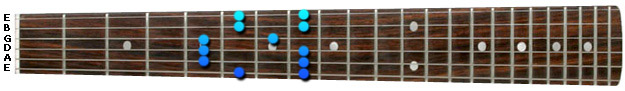• Lessons
• Articles
Tutorial - Pentatonic Scales

# Pentatonic Scales - Position 2

Sean Conklin 456 lessons
Tutorial

## Pentatonic Scales

Now, let's examine the second position.

Position 2 is the position that primarily represents the Major key. So we can apply the same process as we did with Position 1 when we found the minor key on the fretboard.

For an example, let's say I wanted you to play the pentatonic scale in the key of C Major. You could then find the C root note on the Low E string. Then, since we're in a Major key, you would apply the 2nd position starting on the C note (8th fret).

Here is position 2 in G minor (also A# Major):

Position 2Send this to a friend